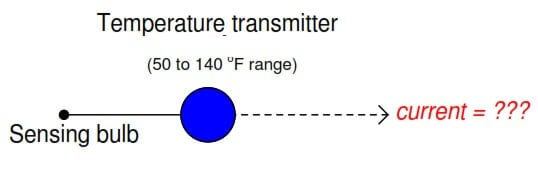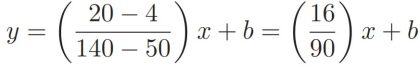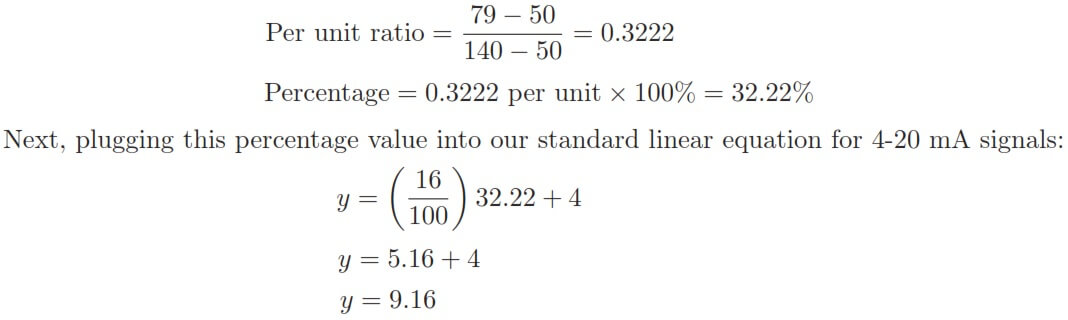# Calculate Temperature Transmitter Output

#### An electronic  temperature transmitter is ranged  50 to 140 degrees  Fahrenheit and  has  a 4-20 mA output  signal.   Calculate  the  current output  by this  transmitter if the  measured  temperature is 79 degrees Fahrenheit.We are solving this problem using standard 4-20mA conversion Formula. Click here for formula

First, we will set up a linear equation describing  this temperature transmitter’s function:Calculating and substituting the slope (m)  value for this equation, using the full rise-over-run  of the linear function:The y-intercept value will be different  for this example than it was for previous examples,  since the measurement range is not zero-based.  However, the procedure  for finding this value is the same– plug any corresponding  x and  y values into the equation and  solve for b.  In this case, I will use the values of 4 mA for y and 50 o F for x:We may apply the same alternative method of solution to this problem as we did for the flowmeter example:   first  converting  the process  variable  into  a  simple  “per  unit” ratio  or  percentage  of measurement range,  then using  that percentage to calculate current in milliamps.

The  “tricky” aspect  of this example is the fact the temperature measurement range does not begin at zero.

Converting  79 deg F  into  a  percentage  of a  50-140 deg F  range  requires  that we first  subtract the live-zero value, then divide by the span:Again,  we arrive  at the exact  same figure for transmitter output current:  9.16 milliamps  at a measured temperature of 79 o F.

The  choice to calculate  transmitter current  by first  setting  up  a “customized”  linear  equation for the transmitter in question  or by  converting  the measured  value  into  a percentage  and  using a “standard” linear  equation for current is arbitrary.

Either method  will produce  accurate results, although it could be argued that the “customized equation” approach may save time if many different current values must be calculated.

We can also solve the problem using direct formula. Click here for formula

#### Articles You May Like :

PLC Example with Switches

Flow Meter Turndown Ratio

Fieldbus Transmitter Calibration

Relationship of Temperature Scales

Formula – 4-20ma from percentage

Don't Miss Our Updates
Be the first to get exclusive content straight to your email.
We promise not to spam you. You can unsubscribe at any time.